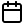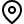## Residual Similarity Based Conditional Independence Test and Its Application in Causal Discovery

### Hao Zhang, Shuigeng Zhou, Kun Zhang, Jihong Guan

[AAAI-22] Main Track
Abstract: Recently, many regression based conditional independence (CI) test methods have been proposed to solve the problem of causal discovery. These methods provide alternatives to test CI of \$x,y\$ given \$Z\$ by first removing the information of controlling set \$Z\$ from \$x\$ and \$y\$, and then testing the independence between two residuals \$R_{x,Z}\$ and \$R_{y,Z}\$. When these residuals are linearly uncorrelated, the independence test between residuals is nontrivial. With the ability to calculate inner product in high-dimensional space, kernel-based methods are usually used to achieve this goal, but still consume considerable time. In this paper, we investigate the independence between two linear combinations under linear non-Gaussian structural equation model. We show that the dependence between two residuals \$R_{x,Z}\$ and \$R_{y,Z}\$ can be captured by the difference between the similarity of \$(R_{x,Z},R_{y,Z})\$ and that of \$(R_{x,Z},R_{r})\$ (\$R_{r}\$ is an independent copy of \$R_{y,Z}\$) in high-dimensional space. With this result, we design a new method called SCIT for CI test, where permutation test is performed to control Type I error rate. The proposed method is simpler yet more efficient and effective than the existing ones. When applied to causal discovery, the proposed method outperforms the counterparts in terms of both speed and Type II error rate, especially in the case of small sample size, which is validated by our extensive experiments on both synthetic and real-world datasets.

## Sessions where this paper appears

• #### Poster Session 4Blue 3

• #### Poster Session 9Blue 3